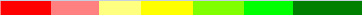B4J Question Paint a label based on its value

Discussion in 'B4J Questions' started by jroriz, Dec 3, 2018.

Tags:
1. Hi.

Is there a better way to acomplish this?
I need to paint a label, based on the value, from light red to solid green, passing through the yellow.

My solution:
Code:
' Use: myLabel.Style = Paint(mylabel.text)
Sub Paint(value As Double) As String

Dim rgb As String

If value > 90 Then rgb = "0,128,0"

If value > 80 And value < 91 Then rgb = "0,255,0"

If value > 60 And value < 81 Then rgb = "128,255,0"

If value > 40 And value < 61 Then rgb = "255,255,0"

If value > 20 And value < 41 Then rgb = "255,255,128"

If value > 10 And value < 21 Then rgb = "255,128,128"

If value < 11 Then rgb = "255,0,0"

Return \$"-fx-Font-size: 18px; -fx-Font-weight: bold; -fx-background-color: rgb(\${rgb});"\$
End Sub
Could be a range like this:Last edited: Dec 3, 2018
2. 1. Don't set the Style property directly. Use CSSUtils.SetBackgroundColor or B4XView.Color.

2. Your code doesn't look 100% correct. What is the expected value for 20.5?

3. I opted for the style because I need to set bold as well.
The expected value for 20.5 can be either 20.5 or 20. You mentioned this because there is an implicit conversion from string to double?

4. Set properties with CSSUtils. Otherwise you will overwrite existing properties.

It will be True in both tests:
Code:
If value > 20 And value < 41 Then rgb = "255,255,128"
If value > 10 And value < 21 Then rgb = "255,128,128"
It will of course work however it looks like a programming mistake.

5. Now I see...
Correct code.

Code:
If value > 90 Then rgb = "0,128,0"

If value > 80 And value < 91 Then rgb = "0,255,0"

If value > 60 And value < 81 Then rgb = "128,255,0"

If value > 40 And value < 61 Then rgb = "255,255,0"

If value > 20 And value < 41 Then rgb = "255,255,128"

If value > 9 And value < 21 Then rgb = "255,128,128"

If value < 10 Then rgb = "255,0,0"

Last edited: Dec 3, 2018
6. You could use a select statement, it makes the code shorter to write and I think clearer.( but that's just personal choice)

Code:
Dim rgb As String ="255,0,0"

Select True

Case (value > 90): rgb="0,128,0"

Case (value > 80): rgb="0,255,0"

Case (value > 60): rgb="128,255,0"

Case (value > 40): rgb="255,255,0"

Case (value > 20): rgb="255,255,128"

Case (value >=10): rgb="255,128,128"

End Select

Last edited: Dec 4, 2018
jroriz and Erel like this.